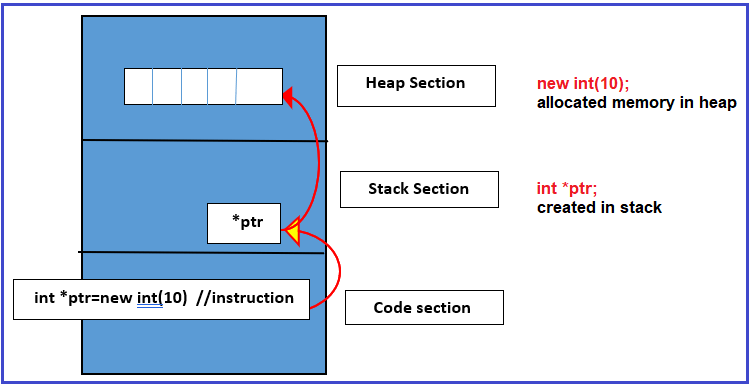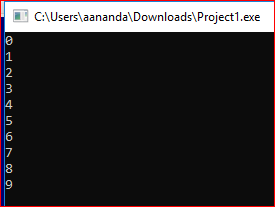# Dynamic Memory Allocation in C++

## Dynamic Memory Allocation in C++ with Examples:

In this article, I am going to discuss Dynamic Memory Allocation in C++ Language with examples. Please read our previous articles, where we discussed Why do we need Pointers in C++ with examples. At the end of this article, you will understand how heap memory is accessed using pointers.

##### Dynamic Memory Allocation in C++ using Pointers:

Allocating memory in the heap section is happening at runtime, not at the compile-time, and hence Allocating a heap memory is called Dynamic memory Allocation.

Let’s see how to do it. Generally, we won’t allocate a single memory in the heap as you already know the complexity or circus we usually do when we use pointers. So only to allocate a block of memory we are going to use heap memory means generally for arrays we usually do dynamic memory allocation.

int array; //get allocating in the stack; but to allocate in heap we need to use ‘new’ operator;

int *ptr= new int(10); this will allocate memory in heap. new int(10); allocated memory in the heapNote: memory allocated in the stack is automatically deallocated once the variable is out of scope but the same is not true for memory allocated in the heap. We need to explicitly deallocate memory using the delete operator in C++. For the above Example:

delete []ptr;

ptr=null;

##### Example Program to Show Dynamic Memory Allocation in C++ using Pointer:
```#include<iostream>
using namespace std;
int main() {
int *ptr=new int(10);
for(int i=0;i<10;++i){
*(ptr+i)=i;
}
for(int i=0;i<10;++i){
cout<<*(ptr+i)<<endl;
}
delete []ptr;
*ptr=NULL;
return 0;
}
```
###### Output:##### Dynamic Memory Allocation in C++ (In Depth):

Heap Memory is accessed dynamically means the memory is allocated dynamically. The size of the memory required in the heap is decided at run time, not at compile time. So mostly when we want to locate memory in heap we allocate arrays, not just one integer or one float or one character, we will have an array of characters. So let us see the difference between when the memory is allocated in the stack and when the memory is allocated in the heap.

int A = {1,4,6,3,8};

This array will be created inside the stack. So, when we declare an array like this it will be created in the stack. Then how it is created in heap? So far that we have to declare it as,

int *p ;

p = new int;

Here first we have declared a pointer ‘p’ then this ‘p’ is also created inside the stack. Then next have assigned ‘p’ as new int of the size of five. With the ‘new’ keyword in C++, we will get memory in heap. The address of this array is stored in ‘p’. So, this P will be having the address of the array which is created in heap memory. So, when you take a pointer and whenever you say new, the array will be created in heap. Below is the structure of memory,The ‘new’ means memory is allocated in heap and just declare an array, it is created in the stack. So, whatever the variables we have declared inside the program or main function, all the variables will get the memory inside the stack. When we write new, we will get the memory in heap and that address should be kept in some address variable.

int *p = new int;

This is created in the heap so we can write it like this also. Now one more difference between stack memory and heap memory is that the array is created inside the stack, automatically, then it will automatically get deleted when it is going out of the scope but heap memory will not be getting deleted automatically. It will be there as well as your program is running so if you don’t want it through the program or you wanted it for a limited time then you should also deallocate it, heap memory must be deallocated, this is a very important thing, as we are writing new for allocation then after some time if we do need it then we will write delete []p, as p is an array then e should write this subscript symbol ‘[]’.

We should not assign p to null before deleting the memory. Because the pointer will not be pointing that memory but the memory is still there inside the heap as long as the program is running. So, assume that you have a big program somewhere and function has stopped but the program is running that memory will be as it is.

So, you must deallocate when you’re not using it. Otherwise, if you’re not deleting it then it is called a memory leak problem, which means that memory belongs to a program, and suppose the pointer is not pointing onto that one then it is a memory leak that memory is of no use. There is no pointer and it is not also free.

Now one thing if a pointer is not pointing anywhere then we say a pointer is null. It’s a null pointer. Let us talk about accessibility. We can access elements of stack array or heap array as,

A = 4;

P = 3;

We can even read that value, write the value. We can do anything with this array by using its index. Just like the array name we can access it. So that is the benefit. Once you have a pointer to an array inside the heap, we can access it with the help of a pointer and the pointer can be treated as a name.

##### Program for Dynamic Memory Allocation in C++:
```#include<iostream>
using namespace std;
int main()
{
int size;
cout << "Enter no of ELements:\n";
cin >> size;
int *p = new int[size];

cout << "Eneter Elements: \n";
for (int i = 0; i < size; i++)
{
cin >> p[i];
}

cout << "Elements are: \n";
for (int i = 0; i < size; i++)
{
cout << p[i] << endl;
}

delete[]p;
return 0;
}
```
###### Output: